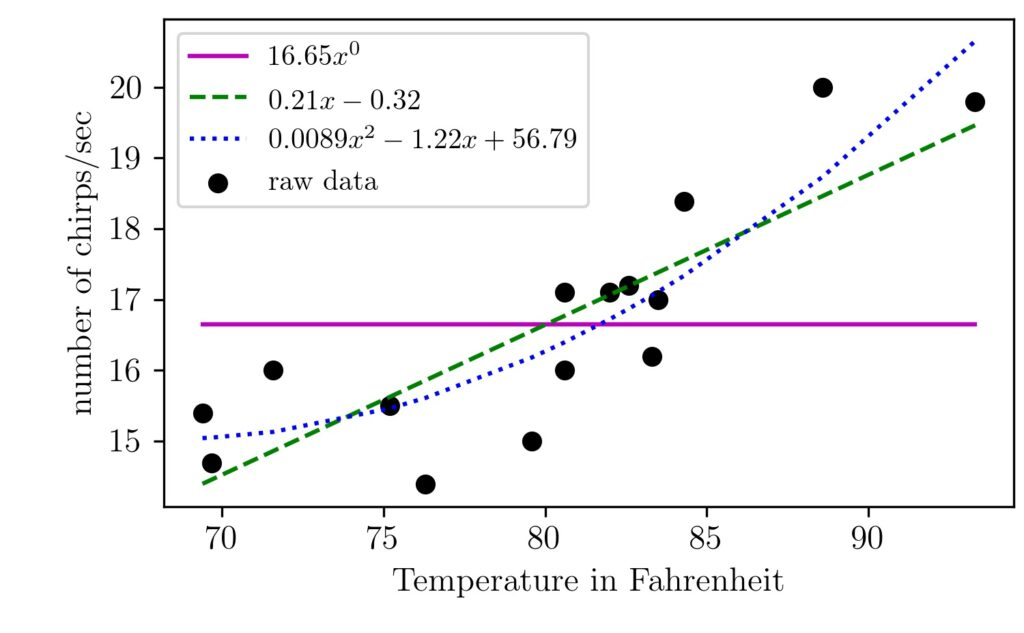## An Intuitive Explanation of Linear Regression

Linear regression is one of the algorithm machine learning enthusiasts start to learn first. In this article, l will walk you through the linear regression intuition. We will implement the basic form of it without using any machine learning packages. The main philosophy of supervised machine learning algorithms is to learn from data. The algorithms …